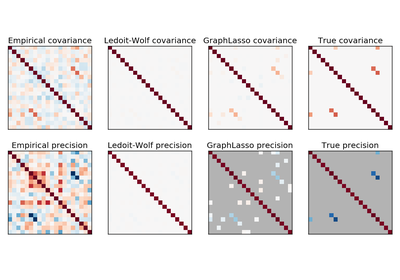# `sklearn.datasets`.make_sparse_spd_matrix¶

`sklearn.datasets.``make_sparse_spd_matrix`(dim=1, alpha=0.95, norm_diag=False, smallest_coef=0.1, largest_coef=0.9, random_state=None)[source]

Generate a sparse symmetric definite positive matrix.

Read more in the User Guide.

Parameters: dim : integer, optional (default=1) The size of the random matrix to generate. alpha : float between 0 and 1, optional (default=0.95) The probability that a coefficient is zero (see notes). Larger values enforce more sparsity. random_state : int, RandomState instance or None, optional (default=None) If int, random_state is the seed used by the random number generator; If RandomState instance, random_state is the random number generator; If None, the random number generator is the RandomState instance used by np.random. largest_coef : float between 0 and 1, optional (default=0.9) The value of the largest coefficient. smallest_coef : float between 0 and 1, optional (default=0.1) The value of the smallest coefficient. norm_diag : boolean, optional (default=False) Whether to normalize the output matrix to make the leading diagonal elements all 1 prec : sparse matrix of shape (dim, dim) The generated matrix.

Notes

The sparsity is actually imposed on the cholesky factor of the matrix. Thus alpha does not translate directly into the filling fraction of the matrix itself.

## Examples using `sklearn.datasets.make_sparse_spd_matrix`¶Sparse inverse covariance estimation